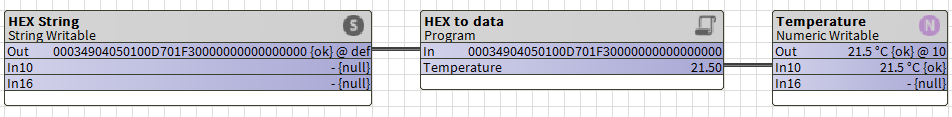1. Innon Knowledge Base
2. Tridium Niagara
3. Java, Program object quick bits

# HEX payload... Extract more Numeric data from it (Getting deeper into Java)

You might have seen our other article on how to convert an HEX string to a numeric value, HERE.

Although very useful, it requires you to slice the HEX string with Niagara blocks to extract the part containing your values. Considering that usually the HEX string comes from a payload containing all sort of information, from temperature to humidity, pulse counters, CRC, etc... How do we make our Java code a bit smarter?

Please refer back to the article linked above if you are new to Program Objects and you have any doubt on how to drag a Program Object, how to create your Pin Slots and how to compile the code (start easy).

For our code I am going to take a real payload example from one of our Enless sensors, which was connected over LoRa WAN.

The way we are going to approach this is by converting the HEX string into an array of bytes. So a string like "00034904050100D701F30000000000000000" will be separated into an array of individual bytes, which would look like        [D7]  [F3]        .

Now that I have my array of bytes, I can check the Enless documentation (in this case there is an Excel sheet where I can check the meaning of each byte for each sensor). The document tells me that bytes 6 and 7 ( [D7]) are my temperature (decimal value 215, divide by 10 is 21.5 degrees Celsius), and that bytes 8 and 9 ( [F3]) are my humidity (decimal value 499, divide by 10 is 49.9%).

In Java then, I can extract those bytes and create a new "sliced" array with just the 2 bytes I need.

I then need to create a formula that puts together those 2 bytes and returns me an integer number. I had to create 2 formulas: one that just puts together the 2 bytes to return an unsigned 16 bits integer, and then a second one that checks for bit 15 and converts the number into a signed integer. Depending on the data you need to extract (i.e. temperature is signed, CO2 level would be unsigned) you can use the right one for you.

Note that I had to always filter the data with "AND FF". The reason is Java uses signed byte notation on byte arrays (-128 to 127 instead of 0 to 255), so filtering it by AND each bit with FF I use it as unsigned.

Enough talking, let's have a look at the code, which contains all of the above, and is extracting just the temperature. The code comments (line starting with "// ") will give an indication on what that part of the code does:

`public void onStart() throws Exception{  // start up code here}public void onExecute(){// Transform the HEX string into an array of bytesString Lora16 = getIn(); int len = Lora16.length();    byte[] decoded = new byte[len / 2];    for (int i = 0; i < len; i += 2) {      decoded[i / 2] = (byte) ((Character.digit(Lora16.charAt(i), 16) << 4) + Character.digit(Lora16.charAt(i+1), 16));    }// slice from index 6 to index 8, and then run the formula to put the 2 bytes together into a signed valuebyte[] sliced = Arrays.copyOfRange(decoded, 6, 8);double temperature = readInt16LE(sliced) / 10d;setTemperature(temperature);}public void onStop() throws Exception{  // shutdown code here}// Code to create unsigned data from 2 bytespublic long readUInt16LE(byte[] bytes) {    long value = ((bytes & 0xFF) << 8) + (bytes & 0xFF);    return value & 0xFFFF;}// Code checking bit 15 of my unsigned data and transform it into a signed valuepublic double readInt16LE(byte[] bytes) {    double ref = readUInt16LE(bytes);    return ref > 0x7fff ? ref - 0x10000 : ref; }`

You need to create 2 Slot Pins for this block:

Slot "In", type "Baja - String", flags "Execute On Change" and "Summary"

Slot "Temperature", type "Baja - Double", flags "Readonly" and "Summary"

Using it on a wire sheet, you can see how it avoids the need of using additional blocks to slice your string and would return you directly the values you are after: